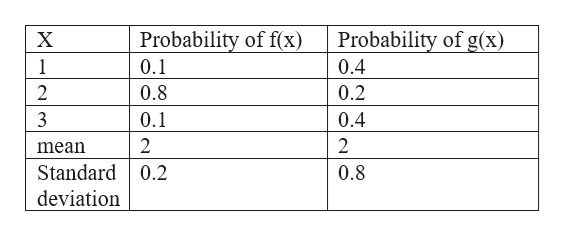# Consider two discrete probability distributions with the same sample space and the same expected value. Are the standard deviations of the two distributions necessarily equal? Explain. No, the individual probabilities may differ in a way that produces the same expected value but a different standard deviation.Yes, because both distributions have the same sample space, they will have the same standard deviation as well.     No, the standard deviations of two different discrete probability distributions are never equal.Yes, because both distributions have the same expected value, they will have the same standard deviation as well.

Question

Consider two discrete probability distributions with the same sample space and the same expected value. Are the standard deviations of the two distributions necessarily equal? Explain.

No, the individual probabilities may differ in a way that produces the same expected value but a different standard deviation.Yes, because both distributions have the same sample space, they will have the same standard deviation as well.     No, the standard deviations of two different discrete probability distributions are never equal.Yes, because both distributions have the same expected value, they will have the same standard deviation as well.
check_circleExpert Solution
Step 1

The correct option is
No, the individual probabilities may differ in a way that produces the same expected value but a different stan...help_outlineImage TranscriptioncloseProbability of f(x) Probability of g(x) X 1 0.1 0.4 0.2 2 0.8 0.4 0.1 2 2 mean Standard 0.2 0.8 deviation fullscreen

### Want to see the full answer?

See Solution

#### Want to see this answer and more?

Solutions are written by subject experts who are available 24/7. Questions are typically answered within 1 hour*

See Solution
*Response times may vary by subject and question
Tagged in

### Basic Probability